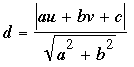SEARCH HOMEMath Central Quandaries & QueriesQuestion from liza, a student: Find the equation of the circle of radius squareroot 26 tangent to the line 5x+y=13 and having its center on the line 3x+y+7=0.Liza,

For this problem you should first know the formula that gives the distance from a point (u,v) to a line written in the form ax + by + c = 0: In the equation of the line, replace x by u and y by v, giving you some number. If that number is positive, call it n; if it is negative, then drop the minus sign and call the positive number n. Next, find the square root of a2 + b2 (where a and b are the coefficients of x and y in the equation of the line); call that number r. The distance from (u,v) to the line is then n/r. This formula isFor example, if the point is P = (1,2) and the line is 3x + 4y + 5 = 0,

n = 3 × 1 + 4 × 2 + 5 = 16;

r = sqrt(32+ 42) = sqrt(25) = 5.

The distance from P to 3x + 4y + 5 = 0 is therefore 16/5 (= 3.2).

In your problem the line is 5x + y - 13 = 0, and you are told that (u,v) is placed on the other line so that its distance from the first line is sqrt(26). That means you must find the unknown u and v by solving an equation. The fact that (u,v) is on the line 3x + y + 7 = 0 tells you that if you set u = x, then v = y = -3x - 7. So for n you have the positive value of 5x + (-3x - 7) - 13, and for r you have sqrt(52 + 1). Set the quotient n/r equal to the desired value of sqrt(26), and solve for x. That allows you to find y, which gives you the centre of your circle and, from that, the equation of your circle.

ChrisMath Central is supported by the University of Regina and The Pacific Institute for the Mathematical Sciences.